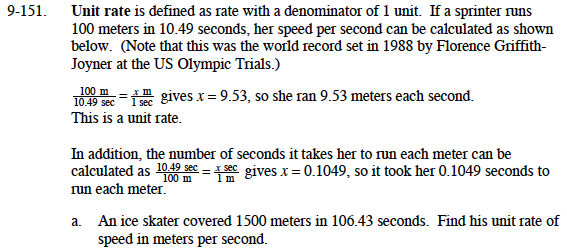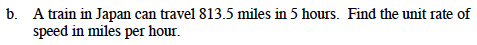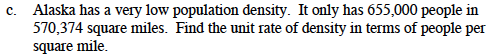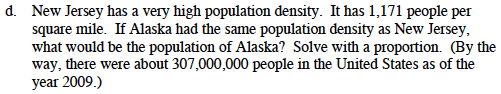### Home > MC1 > Chapter 9 > Lesson 9.3.3 > Problem9-151

9-151.$\frac{1500}{106.43}=\frac{x}{1}$

106.43x = 1500

x = about 14 meters per secondFollow the steps in part (a) and in the example above.About 1.15 people per square mile.$\frac{1,171\text{ people}}{1\text{ sq mile}}=\frac{x \text{ people}}{570,374\text { sq miles}}$